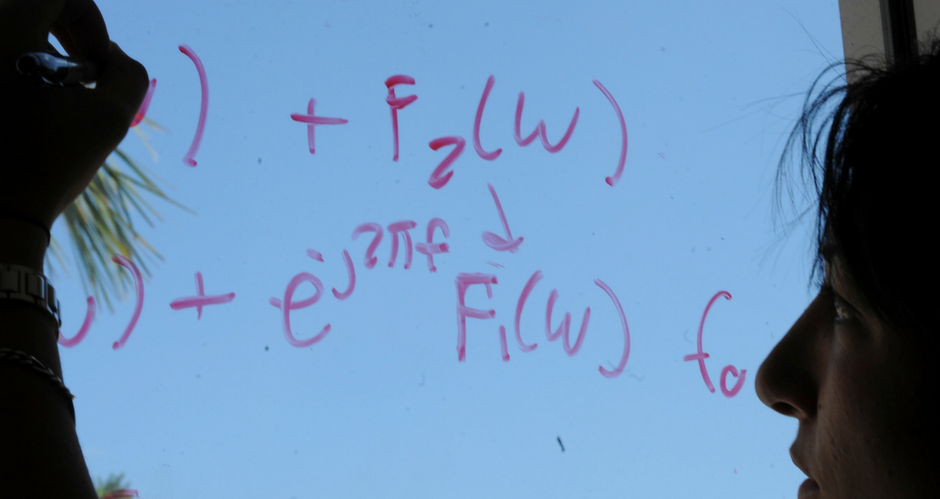## Mathematics and System Engineering Faculty Publications

### Monotone iterations for differential equations with a parameter

Article

#### Publication Title

Journal of Applied Mathematics and Stochastic Analysis

#### Abstract

Consider the problem {y′(t)=f(t,y(t),λ),t∈J=[0,b],y(0)=k0,G(y,λ)=0. Employing the method of upper and lower solutions and the monotone iterative technique, existence of extremal solutions for the above equation are proved.

273

278

#### DOI

10.1155/S1048953397000348

1997

COinS